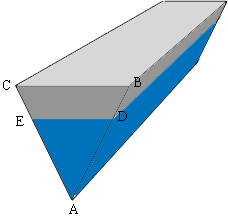SEARCH HOMEMath Central Quandaries & QueriesQuestion from tushar: a barge of triangular cross section is 20m long 12 m wide and 6m deep.its floats in SW at a draft if 4m find its displacementTushar,

In my diagram I have shaded blue the portion of the barge that is below the surface of the water. The shape of this portion of the barge is a triangular prism and the volume of a prism is the area of the base times the height.The displacement is the volume of water that the barge displaces so in this case it is the volume of the triangular prism with base the triangle $ADE$ and height 20 m. The area of the triangle $ADE$ is the length of its base $DE$ times its height of 4 m. Triangles $ABC$ and $ADE$ are similar. What is the length of $DE?$

PennyMath Central is supported by the University of Regina and the Imperial Oil Foundation.